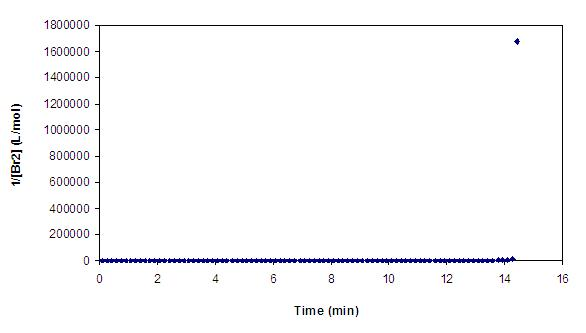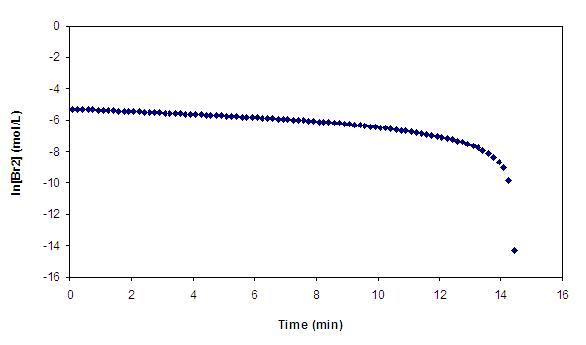# 5.1: Determining Reaction Order

$$\newcommand{\vecs}{\overset { \rightharpoonup} {\mathbf{#1}} }$$ $$\newcommand{\vecd}{\overset{-\!-\!\rightharpoonup}{\vphantom{a}\smash {#1}}}$$$$\newcommand{\id}{\mathrm{id}}$$ $$\newcommand{\Span}{\mathrm{span}}$$ $$\newcommand{\kernel}{\mathrm{null}\,}$$ $$\newcommand{\range}{\mathrm{range}\,}$$ $$\newcommand{\RealPart}{\mathrm{Re}}$$ $$\newcommand{\ImaginaryPart}{\mathrm{Im}}$$ $$\newcommand{\Argument}{\mathrm{Arg}}$$ $$\newcommand{\norm}{\| #1 \|}$$ $$\newcommand{\inner}{\langle #1, #2 \rangle}$$ $$\newcommand{\Span}{\mathrm{span}}$$ $$\newcommand{\id}{\mathrm{id}}$$ $$\newcommand{\Span}{\mathrm{span}}$$ $$\newcommand{\kernel}{\mathrm{null}\,}$$ $$\newcommand{\range}{\mathrm{range}\,}$$ $$\newcommand{\RealPart}{\mathrm{Re}}$$ $$\newcommand{\ImaginaryPart}{\mathrm{Im}}$$ $$\newcommand{\Argument}{\mathrm{Arg}}$$ $$\newcommand{\norm}{\| #1 \|}$$ $$\newcommand{\inner}{\langle #1, #2 \rangle}$$ $$\newcommand{\Span}{\mathrm{span}}$$$$\newcommand{\AA}{\unicode[.8,0]{x212B}}$$

Kinetics are used to study of the rate of the reaction and how factors such as temperature, concentration of the reactants, and catalysts affect it. The reaction order shows how the concentration of reactants affects the reaction rate. Determining the reaction rate also helps determine the reaction mechanism. For the reaction $$\ce{A \rightarrow B}$$ the rate is given by

$\text{rate}=[\text{A}]^x[\text{B}]^y \nonumber$

The overall rate is the sum of these superscripts.

## Introduction

Although there is an infinite variety of rates laws possible, three simple reaction orders commonly taught: zeroth, first, and second order.

In zero order reactions, the disappearance of reactants is

$\dfrac{-d[\text{A}]}{dt}= k[\text{A}]^0= k \nonumber$

Its integrated form is

$[\text{A}]=-kt+[\text{A}]_0 \nonumber$

In first order reactions, the disappearance of reactants is

$\dfrac{-d[\text{A}]}{dt}=k[\text{A}]^1 \nonumber$

The integrated form is

$[\text{A}]=[\text{A}]_0 e^{-kt} \label{1st}$

In second order reactions with two reactant species, the rate of disappearance of $$\text{A}$$ is

$\dfrac{-d[\text{A}]}{dt}= k[\text{A}][\text{B}] \nonumber$

The integrated form is

$\dfrac{1}{[\text{B}]_0-[\text{A}]_0}\ln \dfrac{[\text{B}][\text{A}]_0}{[\text{B}]_0[\text{A}]}=kt \label{2nd}$

when $$[\text{B}]_0>[\text{A}]_0$$.

When $$[\text{B}]_0>>[\text{A}]_0$$, then $$[\text{B}] \approx [\text{B}]_0$$ and Equation $$\ref{2nd}$$ becomes

$\dfrac{1}{[\text{B}]_0-[\text{A}]_0}\ln \dfrac{[\text{B}][\text{A}]_0}{[\text{B}]_0[\text{A}]} \approx \dfrac{1}{[\text{B}]_0}\ln \dfrac{[\text{A}]_0}{[\text{A}]}=kt \nonumber$

or

$[\text{A}] = [\text{A}]_0 e^{-[\text{B}]kt} \nonumber$

This functional form of the decay kinetics is similar ot the first order kinetics in Equation $$\ref{1st}$$ and the system is said to operate under pseudo-first order kinetics.

In second order reactions with one reactant, the disappearance of reactants is

$\dfrac{-d[\text{A}]}{dt}= k[\text{A}]^2 \nonumber$

The integrated form is

$\dfrac{1}{[\text{A}]}= kt+\dfrac{1}{[\text{A}]_0} \nonumber$

## Graphical Method

The graphical method makes use of the concentrations of reactants. It is most useful when one reactant is isolated by having the others in large excess. A series of standards is used to make a Beer's Law plot, the best-fit equation of which is used to determine the concentration of the isolated reactant. To test for the order of reaction with regard to that reactant, three plots are made. The first is concentration of the isolated reactant versus time. The second is of inverse concentration versus time, while the third is of the natural log of concentration versus time. These graphs, respectively, show zero, first, and second order dependence on the specific reactant. The graph that is most linear shows the order of the reaction with regard to that reactant.

$\ce{ CH_3COCH_3 + Br_2 + H^+ \rightarrow CH_3COCH_2Br + HBr} \nonumber$

For example, in the acid-catalyzed bromination of acetone, the concentration of bromine is isolated and measured, while the concentrations of the hydrogen ion and acetone are not. The three graphs from a run are below. The graph of concentration versus time is the one that is most linear, so the order of the bromination reaction with respect to bromine is zero. The slope of the best-fit line gives $$-k_{obs}$$. In theory, the other reactants could be isolated like bromine was, but the data from bromine can be used to determine the reaction order with regard to them as well. It is found by comparing two reactions where only the concentration of the reactant in question is changed. If p is the reaction order with regard to acetone,

$p = \dfrac{ \log \dfrac{k_{obsII}}{k_{obsI}}}{ \log u } \nonumber$

The observed rate constant at the higher concentration of acetone is $$k_{obsII}$$, while $$k_{obsI}$$ is the observed rate constant at the lower concentration of acetone. The ratio of the higher concentration to the lower concentration is given by u. The process is repeated for the hydrogen ion.## Mathematical Method

The mathematical method is useful when the means to graph are not available. It is essentially determining the slope of the plot that "linearizes the data". This requires plotting concentration versus time data. As in the graphical method, the inverse and natural log of the concentration must be calculated. A linear plot has a constant slope, so the slopes calculated from two pairs of adjacent points should be the same.Take three consecutive points from the concentration versus time data. Calculate $$\dfrac{\Delta y}{\Delta x}$$ for the first and second points. The concentration is the $$y$$ value, while time is the $$x$$ value. Do the same for the second and third point. If the reaction is zero order with regard to the reactant, the numbers will be the same. If not, then calculate the slope for the inverse concentration versus time data or natural log of the concentration versus time data.

 [Bromine] (M) Time (min) 0.00349 4.25 0.00344 4.42 0.00339 4.58

The slope of the first two points is

$m= \dfrac{0.00344\,\text{M} - 0.00349\,\text{M}}{4.42\, \text{min}-4.25\, \text{min}} \nonumber$

or

$m= -2.9 \times 10^{-4}\dfrac{\text{M}}{\text{min}} \nonumber$

The slope of the second two points is

$\dfrac{0.00476\, \text{M}-0.00479\,\text{M}}{4.58 \,\text{min}-4.42\, \text{min}} \nonumber$

or

$-3.1 \times 10^{-4}\dfrac{\text{M}}{\text{min}} \nonumber$

These are approximately the same, so the bromine depletion follows zero order kinetics.

Another method uses half lives. For a zero-order reaction,

$t_{1/2}=\dfrac{[\text{A}]_0}{2k} \nonumber$

For a first-order reaction,

$t_{1/2}=\dfrac{\ln 2}{k} \nonumber$

For a second-order reaction,

$t_{1/2}=\dfrac{1}{[\text{A}]_0k} \nonumber$

If an increase in reactant increases the half life, the reaction has zero-order kinetics. If it has no effect, it has first-order kinetics. If the increase in reactant decreases the half life, the reaction has second-order kinetics.

## References

1. Chang, Raymond. Physical Chemistry for the Biosciences. 1st. Herndon, VA: University Science Books, 2005. 312-319. Print.
2. Hope College. Physical Chemistry Lab I Laboratory Manual. Holland, MI, 2011. D2-2 and D2-3.